# Wave Walker DSP

## DSP Algorithms for RF Systems

DSP for Beginners: Simple Explanations for Complex Numbers! The second edition includes a new chapter on complex sinusoids.

Easy Guide to Logarithms and Decibels
July 20, 2022

#### Introduction

Logarithms are confusing! This blog explains what logarithms are, how they are used, and gives two easy equations to convert between decibels and linear units.

Check out these other blogs on DSP math:

#### What's a Base?

A number can be represented by a base raised to an exponent. For example, the number 100 can be written as

(1)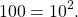The number 10 is the base and the exponent is 2. Here are more examples:

(2)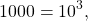(3)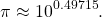The base can be changed to other numbers. For example, base 2:

(4)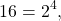(5)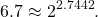The base can also be Euler’s number e,

(6)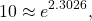(7)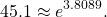#### Why Logarithms?

The base of a logarithm is written as a subscript. For example, a base 10 logarithm is written as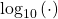, a base 2 logarithm is written as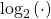, and a natural logarithm may be written as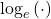or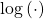.

Logarithms are used to represent numbers by their exponent. Logarithms act as if a number is represented by a base raised to an exponent, and then they calculate that exponent.

From (1), (2), (5) and (7),

(8)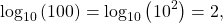(9)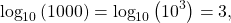(10)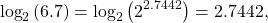(11)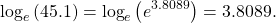#### Linear to Decibels

Engineers commonly use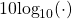to transform linear numbers into units of decibels,

(12)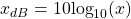which can then be transformed back into linear units through

(13)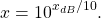Here are some examples using (12) and (13).

Example 1:

(14)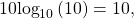(15)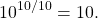Example 2:

(16)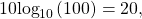(17)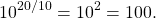Example 3:

(18)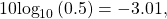(19)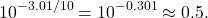Example 4:

(20)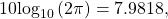(21)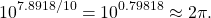#### Conclusion

Numbers can be represented by a base number raised to an exponent. Logarithms are useful in representing the exponents of base numbers. Engineers use 10log10() to convert linear units into decibels.

Check out these other blogs on DSP math:

God, the Lord, is my strength; he makes my feet like the deer’s; he makes me tread on my high places. Habakkuk 3:19

This website participates in the Amazon Associates program. As an Amazon Associate I earn from qualifying purchases.